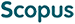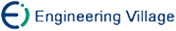李鸣鹏, 邹兆年, 高 宏, 赵正理. 不确定图上期望最短距离的计算[J]. 计算机研究与发展, 2012, 49(10): 2208-2220.
 引用本文: 李鸣鹏, 邹兆年, 高 宏, 赵正理. 不确定图上期望最短距离的计算[J]. 计算机研究与发展, 2012, 49(10): 2208-2220.Li Mingpeng, Zou Zhaonian, Gao Hong, Zhao Zhengli. Computing Expected Shortest Distance in Uncertain Graphs[J]. Journal of Computer Research and Development, 2012, 49(10): 2208-2220.
 Citation: Li Mingpeng, Zou Zhaonian, Gao Hong, Zhao Zhengli. Computing Expected Shortest Distance in Uncertain Graphs[J]. Journal of Computer Research and Development, 2012, 49(10): 2208-2220.## Computing Expected Shortest Distance in Uncertain Graphs

• 摘要: 研究了不确定图上的最短距离问题,提出了期望最短距离的概念,证明了该问题不存在多项式时间的算法.为了解决该问题,使用了随机采样技术获得不确定图的一些可能世界,在每个可能世界上计算有穷的最短距离,最后计算出平均值作为期望最短距离的估计值.为提高计算效率,使用了过滤条件来减少采样过程中采样的边数从而加快随机采样.在此基础上,提出了一种基于对称变量的、无偏的随机采样近似算法,并证明了与直接随机采样方法相比,该方法在不增加时间开销的同时能减小采样方差.通过真实数据上的实验表明,提出的算法在时间开销和采样方差上均明显好于直接随机采样方法.

Abstract: This paper focuses on the shortest distance problem in uncertain graphs, which we call expected shortest distance problem. We analyze the complexity of this problem and prove that there is no polynomial time algorithm for it. To solve this problem, we utilize random sampling methods to acquire some possible worlds of the uncertain graph, then compute the shortest distance on each and estimate expected shortest distance with the average value of the finite ones. To improve efficiency, we propose two pruning techniques, which allow us to terminate a random sampling process faster. Furthermore, considering that different sampling orders of edges do not influence the result of sampling, but will determine the number of edges to be sampled in a sampling process, we propose two sampling orders for edges to reduce the number of edges sampled in each random sampling process. Then, we propose an approximation algorithm based on random sampling using antithetic variables which is an unbiased estimator for expected shortest distance and prove that it outperforms direct random sampling in both efficiency and sampling variance, while the latter one is the key criteria for evaluating the quality of an unbiased estimator. Our experiments on real uncertain graphs of proteinprotein networks demonstrate the efficiency and accuracy of our algorithm./下载:  全尺寸图片 幻灯片
• 分享
• 用微信扫码二维码

分享至好友和朋友圈